We analyze the role of architecture in shaping the inductive bias of deep classifiers, by introducing the concept of Neural Anisotropy Directions (NADs) : A sequence of vectors that encapsulate the directional inductive bias of an architecture and encode its preference to separate the input data based on some particular features.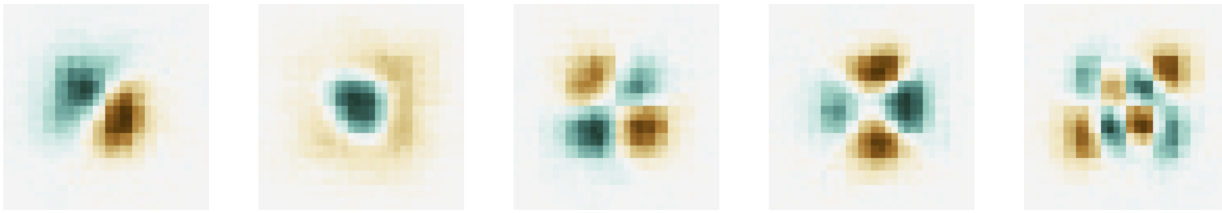Once you are given a finite number of samples, it is usually very easy to find many models that can perfectly classify them, but it is much harder to find a model which can also generalize. To find this model, a machine learning algorithm needs to exploit its so called inductive bias: a set of a priori assumptions about the world that allows it to identify the solution which is most likely to generalize. In deep learning, one of the main sources of inductive bias is the choice of a network's architecture. But, although we know which networks work well in practice, we still do not really understand how this bias influences their behaviour.

In this blog post, we try to give an intuitive explanation on how this happens, and illustrate how the interaction between architecture and data distribution shapes the inductive bias of a neural network. An interaction that allows a neural network to go from merely memorizing the training data to learning the perfect classifier.

We will show this through an embarrasingly simple experiment: training neural networks used in practice to classify a linearly separable distribution. Surprisingly, though, even in this setup the choice of architecture plays a fundamental role, and although all networks achieve good training accuracy, they do not always manage to generalize. We will see that the role of architecture can be summarized by a sequence of vectors, or neural anisotropy directions (NADs), that rank the preference of an architecture to select certain features of the input data. These NADs can be computed very efficiently, even without training, and we will explain why they are fundamental in generalization in more complex datasets, such as CIFAR-10. We hope that these new intuitions can improve your understanding of DNNs.

## An embarrasingly simple experiment

Deep learning is great! But because we mostly study it on complex problems, like classifying ImageNet, we barely know why it works so well in practice. A proof of how little we know about deep networks is how hard it is to to answer this simple question:

Can a modern deep neural network generalize on any linearly separable task?

If you are a true statistician, you would probably think that the answer is clearly "no": CNNs are very complex models, and therefore, they must overfit. However, if you are familiar with deep learning theory, you might be inclined towards a more affirmative answer, instead. Especially if you have read some works that argue that deep architectures are "biased" towards simple functions, and so are pretty good at learning separable distributions. Even provably so, for heavily overparameterized, but simple architectures .

Reality, though, is a bit more nuanced. And in practice, the answer for most CNN architectures seems to be "it depends". In particular, "it depends on the direction of the discriminative information in the dataset". We observe experimentally that each CNN cannot always solve the problem — they can do it only when the distribution is separable by certain hyperplanes. This is, CNNs have a strong directional inductive bias.

Testing this bias is quite easy. You just need to define a linearly separable distribution in which the data (x,y)\sim\mathcal{D}(v) can be separated using a hyperplane orthogonal to v\in\mathbb{R}^{D}, i.e., x=y\,v + w\quad\text{with}\quad y\in\{-1,+1\}\quad\text{and}\quad w\sim\mathcal{N}(0,\sigma^2(I-vv^T)), and train a neural network on multiple versions of it with different values of v. In our experiments, because we focused on CNNs used in image classification, we decided to use Fourier basis vectors.

The figure on the left shows the test accuracy of a LeNet, trained on this linearly separable problem. Each pixel represents the test accuracy of the network trained and tested on a new \mathcal{D}(v).
Test accuracy  50% v=
v \mathbb{R}^{d-1}

As we can see, the test accuracy on this problem heavily depends on the choice of direction vPlace the cursor over a pixel to see the direction v used for training.. While the naïve logistic regression always achieves near-perfect accuracy, all other CNNs only perform well on some instances of this problem. Even the gigantic DenseNet can only learn a few of these distributions!

Now, note that the differences in performance cannot be due to a higher complexity of the CNNs. If that was the case, then deep networks would always show the same test accuracy, regardless of v. But, instead, we are seeing that they can classify only some distributions, despite them all being related by simple linear transformations.

The answer to why this happens is hidden in the architecture. Indeed, if we remove all pooling layers from these CNNs, and compensate the increase of dimensionality with a larger fully connected layer at the end, we will see that these networks generalize for all Fourier directions vIt is not very surprising that pooling has such a strong effect in the directional inductive bias of an architecture towards Fourier basis vectors. As it is widely known in signal processing, downsampling a signal mixes its information in the spectral domain, i.e., it causes aliasing. For this reason, if the features extracted before a pooling layer contain information in different parts of the spectrum, going through pooling might destroy this information as it aliases with some noise..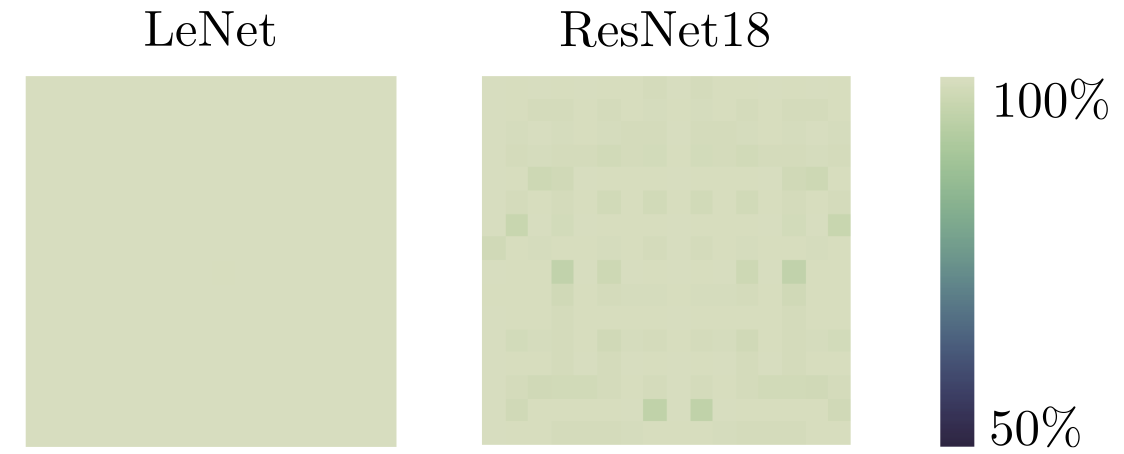But, of course, pooling cannot be the only source of directional inductive bias, as all these CNNs have pooling, yet they generalize on very different sets of distributions. In fact, it looks as if the set of distributions \mathcal{D}(v) that a network can learn is a unique signature of its architecture, as the complex interactions between its different components seems to lead to very different types of generalization patterns.

## Neural anisotropy directions

Training and testing on different instances of \mathcal{D}(v) is not a very scalable procedure to test the inductive bias of an architecture. In fact, there are uncountably many directions in \mathbb{R}^D and we cannot even hope to test them all. But there must be another way to identify which directions a network can learn. Indeed, the directional inductive bias is an intrinsic property of the architecture, so we must be able to observe it even without training. In fact, it seems quite easy to do so.

The key idea here is to stop worrying about training, and just focus on random neural networks, i.e., f_\theta:\mathbb{R}^D\rightarrow\mathbb{R} with \theta drawn from some random weight distributionIn our experiments we drew \theta from the standard weight initalization distribution of the network.; and note that a neural network will achieve good accuracy on \mathcal{D}(v) if its gradient is aligned with vIf the input gradient \nabla_x f_\theta(x) is aligned with a certain direction v in most datapoints, then it means that the decision boundary of the network wil be orthogonal to v.. For this reason, we can ask ourselves, what is the number of networks with this architecture that have their gradient aligned with v, i.e., \mathbb{P}\left(|v^T\nabla_x f_\theta(x)|\geq \eta\right)\leq \frac{v^T\mathbb{E}_\theta \left[\nabla_x f_\theta(x)\nabla^T_x f_\theta(x)\right]v}{\eta^2}, where the upper bound can be simply obtained using Markov's inequality.

This bound is, actually, quite easy to interpret, as it only depends on the eigenvectors of the gradient covarianceGiven any direction v\in\mathbb{R}^D, the bound will be smaller whenever v is mostly aligned with the smallest eigenvectors of \mathbb{E}_\theta \left[\nabla_x f_\theta(x)\nabla^T_x f_\theta(x)\right].. In fact, we can estimate these eigenvectors using Monte Carlo, and performing PCA on a bunch of randomly sampled \nabla_x f_\theta (x). We call the resulting vectors, the neural anisotropy directions or NADs of an architecture.

Visualize the NADs of a LeNet

Spatial domain Frequency domain

What is very surprising is that the NADs fully encapsulate the directional inductive bias of an architecture: even if they are computed using random networks, repeating the same experiment as before but using NADs instead of Fourier vectors, yields a monotonic decay in accuracy of a neural network as we test on higher NAD indicesThe ith NAD is the eigenvector of the gradient covariance associated with its ith largest eigenvalue..

The diversity of NADs of the different networks is quite striking, and it highlights their role as a unique signature of each architecture. It suggests that each architecture uses a unique set of features to classify the data, and that the interactions between architectural elements can create very rich inductive biases. Besides, the fact that we computed NADs using random networks, but still they predict generalization performance after training hints towards a deeper connection between the optimization properties of a neural network and its functional properties. The link between the weight space and the input space on deep neural networks is largely unexplored, and we believe that NADs might give an exciting direction to better explore this connection.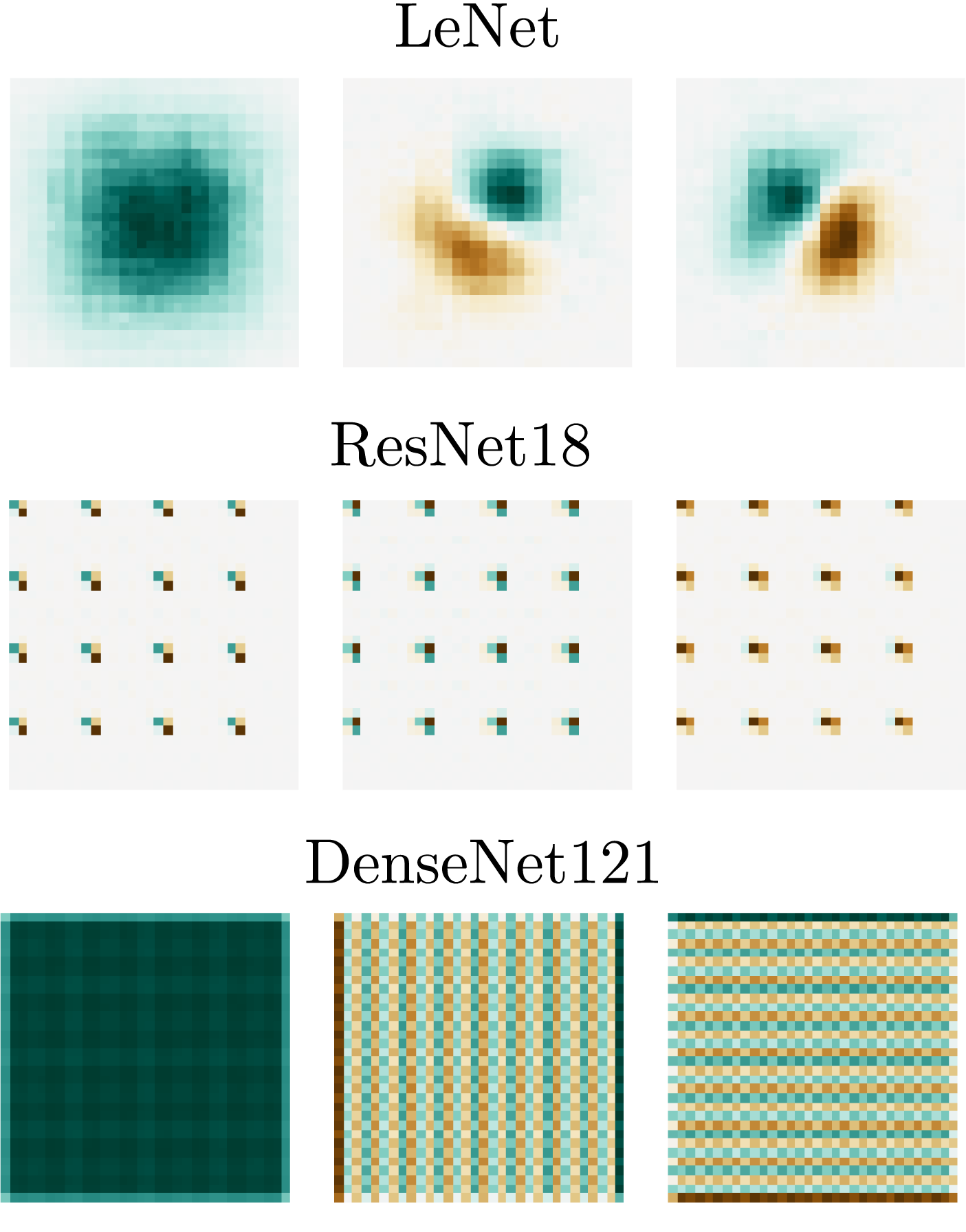We do not have a definite answer, yet, but we believe they do. In fact, some of our most recent experiments suggest that the existence of NADs is necessary for generalization in complex datasets, such as CIFAR-10. We have two main pieces of evidence to believe so.

### Poisoning CIFAR-10

The first piece of evidence suggests that NADs determine the order in which a neural network looks for discriminative information in the training set. Or more informally, a CNN first tries to fit the data based on its projection on the lower NADs, and progressively grows the number of NADs to take into account depending on the training error.

We can actually test this hypothesis by modifying the CIFAR-10 training set. In particular, we can add a spurious feature in a certain NAD component of every CIFAR-10 training image and study what happens to its accuracy on the unmodified test images.

Select training set:

Note that poisoning CIFAR-10 renders its training set linearly separable. However, if a neural network tries to fit the training data using a hyperplane orthogonal to the spurious feature, it will not be able to generalize to the test setIn a sense, this experiment is the opposite to the previous one with \mathcal{D}(v). Before we said that a network generalized nicely if it found the information in v. Now, the network generalizes correctly if it can avoid the information in v..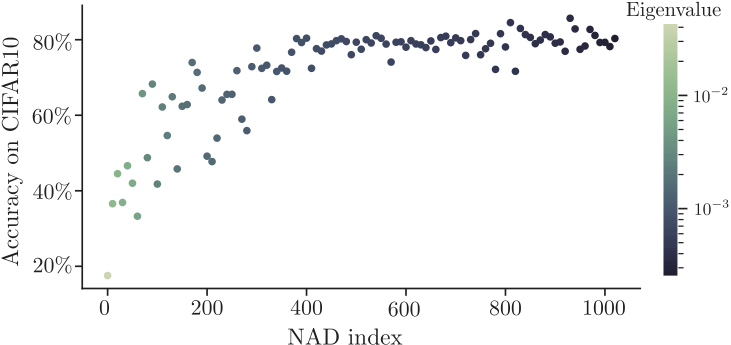Using this setup, we trained a ResNet-18 on multiple versions of the poisoned CIFAR-10 training set where the poisonous feature was placed at different NAD indices.

As we can see, when the dataset is poisoned on the first NAD index, the network fully overfits. But when the spurious feature is placed at the last NAD, the network can generalize. In between these two extremes we see a gradual increase in the test performance. This can only be explained if the network is progressively picking more features from the original CIFAR-10 data, before finding the poisonous signal. This clearly suggests to the existence of an ordered preference of features for CNNs, determined by the NAD basis.

### NADs are necessary for generalization

NADs seem to determine the order of selected features in a dataset, but are they really necessary for generalization? We believe they are, and we actually think that their particular structure is what explains the good performance of most CNNs on image datasetsIt seems that for most CNNs, the NADs are mostly aligned with low spatial frequencies. Natural images are mostly concentrated in this part of the spectrum, and the human visual system is mostly sensitive to information contained on it..

In order to support this hypothesis, we tried a funny experiment whose goal was to investigate the role of NADs as filters of non-generalizing solutions. In particular, we wanted to test the possible positive synergies arising from the alignment of NADs with the generalizing features of the training set.

To do so, we trained multiple CNNs using the same hyperparameters on two representations of CIFAR-10: the original representation, and a new one in which we flipped the representation of the data in the NAD basisThat is, for every sample x in the training and test sets we computed x'= U\,\text{flip}(U^T x), where U represents a matrix with NAD vectors in its columns. . Note that this transformation is a linear rotation of the input space, and it has no impact on the information of the data distribution. In fact, training on both representations yielded approximately 0% training error.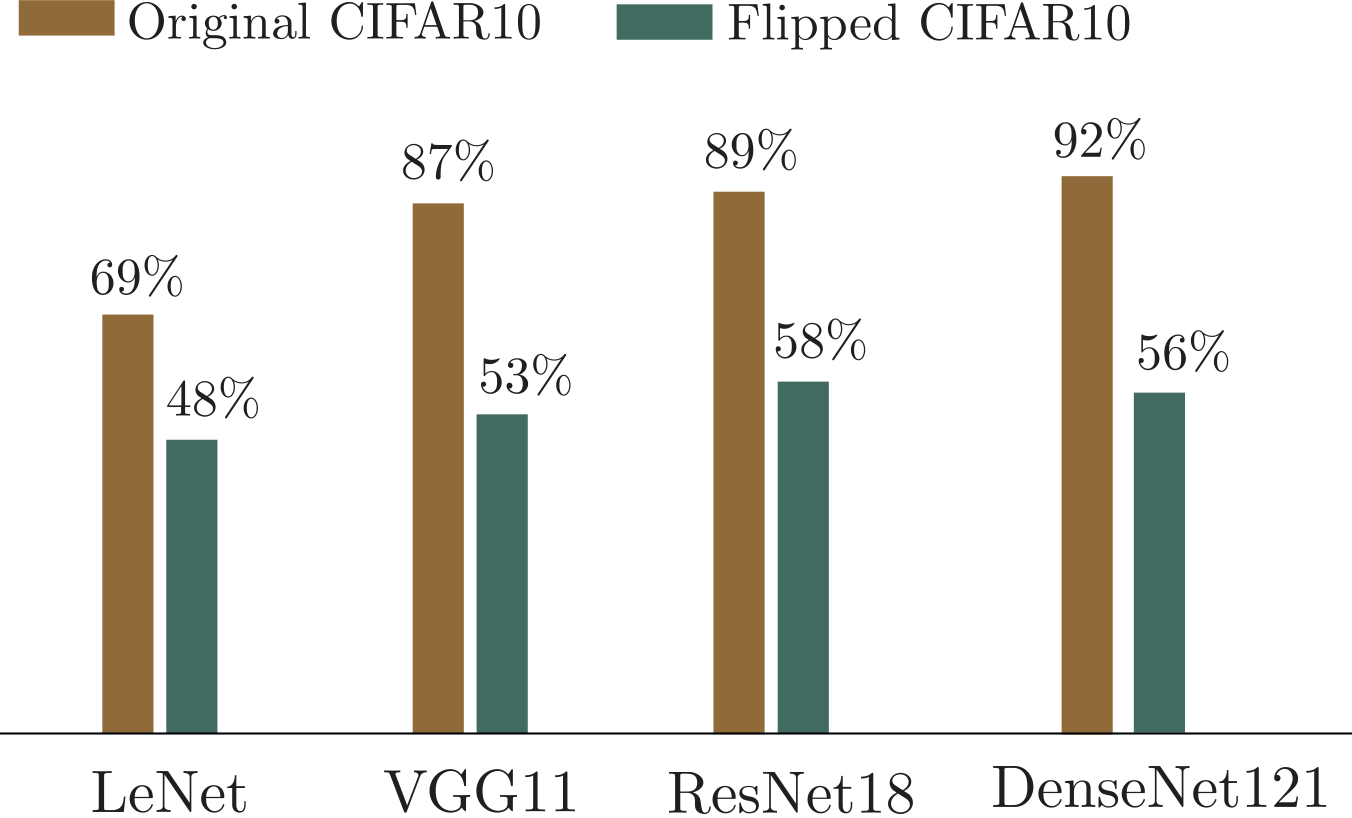The result of these experiments shows that the performance of the networks trained on the flipped datasets is significantly lower than those on the original CIFAR-10. This demonstrates that misaligning the inductive bias of the networks with the dataset makes them prone to overfit.

We see these resutlts as strong supporting evidence that through the years the research community has managed to impose the right inductive biases in deep neural architectures. Letting them filter out spurious and noisy signals and hence being able to solve most standard vision benchmarks.

## Final remarks

In this post, we have described a new type of model-driven inductive bias that controls generalization in deep neural networks: the directional inductive bias. We have seen that this bias is summarized by the NADs of an architecture, which seem to be responsible for the selection of discriminative features by a CNN.

The existence of NADs demonstrates that the full set of inductive biases in deep learning is much richer than it was previously believed. Prior to our work, some researchers highlighted that neural networks could memorize a dataset when there was no generalizable information present in the data . Our experiments, however, complement this observation, and show that most CNNs may prefer memorization over generalization, even when there is some highly discriminative feature in the data. Surprisingly, this phenomenon is not only attributable to some property of the data, but also to the structure of the architecture.

We think there are many possibilities to use NADs in future research and novel applications. For instance, we are very excited about the possibility of using NADs to comprehensively study architectures in deep learning. We have seen that pooling plays an important role in NADs for CNNs, but what about other layers or components in these architectures? And, can we think beyond image data, and use NADs to understand the misterious transformers or the promising GNNs? In general we see NADs as an interesting tool in AutoML, as they can give an explicit framework to align the inductive biases of an architecture, with our a priori knowledge of the task. This gives an exciting path towards the design of new architectures with richer invariances and more robust to adversarial and naturally occuring distribution shifts.

Finally, it is important to note that our results mostly apply to cases in which the data was fully separable, i.e. there was no label noise. And even more specifically, to the linearly separable case. In this sense, it still remains an open problem to understand how the directional inductive bias of deep learning influences neural networks trying to learn non-separable datasets.

### Citation

This blog post is a short version of the NeurIPS 2020 article "Neural Anisotropy Directions". If you want to cite it, please cite the original paper

G. Ortiz-Jimenez, A. Modas, S.M. Moosavi-Dezfooli, and P. Frossard, “Neural Anisotropy Directions,” in Advances in Neural Information Processing Systems 34 (NeurIPS), Dec. 2020

### Acknowledgements

To lighten this article and point the reader only to the most relevant papers, we cited only a subset of the relevant work that we built on. Please refer to the bibliography of the original paper for the complete list.

The article template is due to distill.pub and many formatting styles are inspired from other articles appearing on Distill.